All the solutions provided in McGraw Hill Math Grade 1 Answer Key PDF Chapter 7 Test as per the latest syllabus guidelines.

Read the number sentences. Write the sums.

Question 1.
5 + 7 = ____________
7 + 5 = ____________
5 + 7 = 12
7 + 5 = 12
Explanation:
If we change the pattern of addends the sum doesn’t change,
The sum remains the same.

Question 2.
12 + 6 = ____________
6 + 12 = ____________
12 + 6 = 18
6 + 12 = 18
Explanation:
If we change the pattern of addends the sum doesn’t change,
The sum remains the same.

Question 3.
0 + 8 = ____________
0 + 8 = 8
Explanation:
The sum of 0 and 8 is 8
If we add the zero to a number the number remains the same.

Question 4.
0 + 4 = ____________
4
Explanation:
The sum of 0 and 4 is 4
If we add the zero to a number the number remains the same.

Question 5.
5 + 1 + 2 = ____________
5 + 1 = 6
6 + 2 = 8
Explanation:
The sum of 5 and 1 is 6
and 6 and 2 is 8

Question 6.
3 + 6 + 4 = ____________
6 + 4 = 10
10 + 6 = 16
Explanation:
First we have to form the ten, then we have to add the other number.

Read the problems. Write the missing number.

Question 7.
5 += 9
9 – 5 = ____________
5 + ____________ = 9
5 + 4 = 9
9 – 5 = 4
5 + 4 = 9
Explanation:
To find the missing number in addition we have to subtract

Question 8.
5 +1 = 18
18 – 5 = ____________
5 + ____________ = 18
5 + 13 = 18
18 – 5 = 13
5 + 13 = 18
Explanation:
To find the missing number in addition we have to subtract

Look at each addition sentence. Write another fact with the same sum.

Question 9.
9 + 2 = 11
____________ + ____________ = 11
9 + 2 = 11
2 + 9 = 11
Explanation:
If we change the pattern of addends the sum doesn’t change,
The sum remains the same.

Question 10.
3 + 4 = 7
____________ + ____________ = 7
3 + 4 = 7
4 + 3 = 7
Explanation:
If we change the pattern of addends the sum doesn’t change,
The sum remains the same.

Look at each set of numbers. Write = if they are equal. Write not = if they are not equal.

Question 11.
17 ____________ 17
17 = 17
Explanation:
If the given numbers are equal they are represented with the symbol ‘=’

Question 12.
35 ____________ 30 + 5
30 + 5 = 35
35 = 35
Explanation:
If the given numbers are equal they are represented with the symbol ‘=’

Question 13.
8 + 3 ____________ 12 + 5
11 ≠ 17
Explanation:
If the given numbers are equal they are represented with the symbol ‘=’
If they are not equal we represent with ≠

Question 14.
9 – 3 ____________ 4 + 2
9 – 3 = 6
4 + 2 = 6
6 = 6
Explanation:
If the given numbers are equal they are represented with the symbol ‘=’

Question 15.
8 + 5 = ?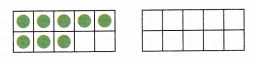10 + 3 = ____________
so 8 + 5 = _____________8 + 5 = 13
10 + 3 = 13
Explanation:
There are 8 green dots in ten frame
we have to add 5 more to get 13

Question 16.
9 + 6 = ?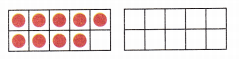10 + 5 = _____________
so 9 + 6 = _____________10 + 5 = 15
9 + 6 = 15
Explanation:
There are 9 red dots in ten frame.
so added 6 to get 15

Count on to add. Count back to subtract.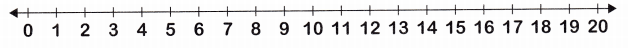Question 17.
4 + 7 = ___________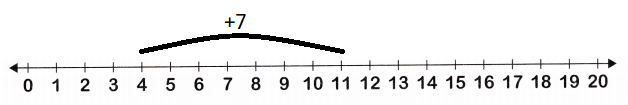Explanation:
The sum of 4 and 7 is 11
4 + 7 = 11

Question 18.
18 – 12 = ___________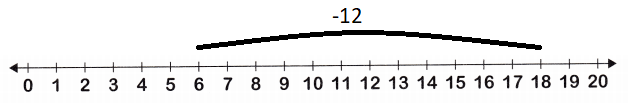Explanation:
The difference of 18 and 12 is 6
18 – 12 = 6

Look at the chart. Write an addition sentence. Then write a subtraction sentence.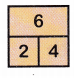Question 19.
__________ + ___________ = ___________
2 + 4 = 6
4 + 2 = 6
Explanation:
If we change the pattern of addends the sum doesn’t change,
The sum remains the same.

Question 20.
__________ – ___________ = ___________
6 – 2 = 4
6 – 4 = 2
Explanation:
The difference of 6 and 2 is 4, 6 and 4 is 2

Add or subtract to solve. Write the sum or difference. Use objects to help.

Question 21.
6play. 2sleep. How manyare there?
6 + 2 = ___________6 + 2 = 8
Explanation:
Number of dogs that play = 6
Number of dogs that sleep = 2
so, there are 8 dogs in all.

Question 22.
Ben has 13He gives 6 to Sara. How manydoes Ben have now?
13 – 6 ___________6that Ben have now